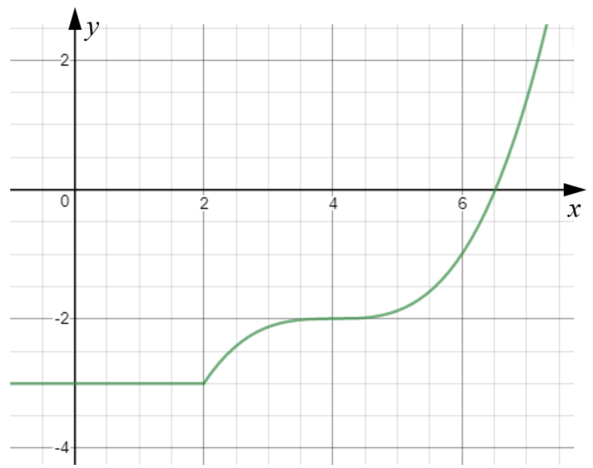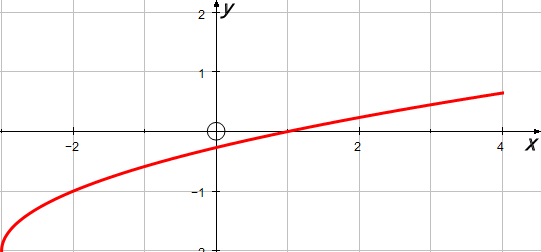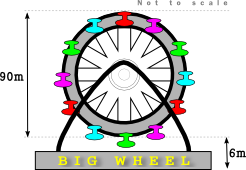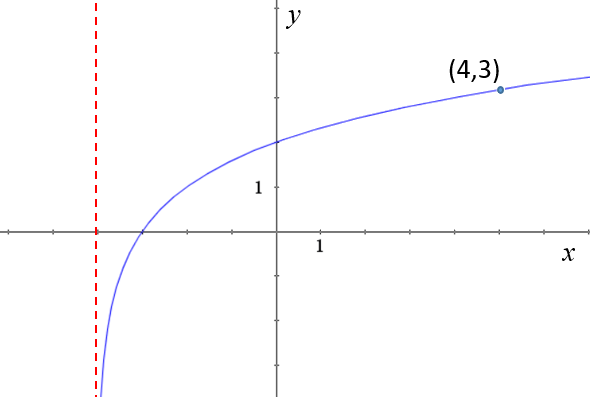# Exam-Style Questions.

## Problems adapted from questions set for previous Mathematics exams.

### 1.

GCSE Higher

$$f(x) = \frac{2x}{5} + 7$$ and $$g(x) = 10x^2 - 15$$ for all values of $$x$$.

Find $$fg(x)$$.

Give your answer in the form $$ax^2 + b$$ where $$a$$ and $$b$$ are integers.

### 2.

GCSE Higher

(a) A function is represented by the following function machine.A number is input into the machine and the output is used as a new input.

If the second output is 53 work out the number that was the first input.

(b) A number is input into the machine and the output produced is the same number. Work out what this number could have been.

(c) Another function machine is shown below.If the Input is 2, the Output is 7.
If the Input is 6, the Output is 27.
Use this information to fill in the two boxes.

### 3.

IGCSE Extended

If $$f(x)=5-4x$$ and $$g(x)=4^{-x}$$ then:

(a) Find $$f(3x)$$ in terms of $$x$$.

(b) Find $$ff(x)$$ in its simplest form.

(c) Work out $$gg(–1)$$ give your answer as a fraction.

(d) Find $$f^{–1}(x)$$, the inverse of $$f(x)$$.

(e) Solve the equation $$gf(x)= 1$$.

### 4.

GCSE Higher

Here is a function machine that produces two outputs, A and B.Work out the range of input values for which the output A is less than the output B.

### 5.

GCSE Higher

The functions $$f$$ and $$g$$ are such that:

$$f(x) = 4x + 3$$ $$g(x) = x^2 - 3$$

(a) Find $$f^{-1}(x)$$

(b) Given that $$gf(x) = 3fg(x)$$, show that:

$$4x^2 + 24x + 33 = 0$$

### 6.

IB Analysis and Approaches

Part of the function of $$y=f(x)$$ is shown in the following diagram.(a) Write down the value of $$f(1)$$.

(b) Write down the value of $$ff(7)$$.

(c) Sketch the graph of $$g(x)$$ if $$g(x) = -f(x)-1$$ on the same set of axes above.

### 7.

IB Analysis and Approaches

The functions $$f$$ and $$g$$ are defined for $$x \in \mathbb{R}$$ by

$$f(x) = ax - b$$, where $$a, b \in \mathbb{Z}$$

$$g(x) = 2x^2 - x + 5$$

Find the values of $$a$$ and $$b$$ such that $$(g \circ f)(x) = 18x^2-51x+41$$

### 8.

IB StandardThe diagram shows the graph of $$y=f(x)$$, for $$-3\le x \le 4$$.

The graph passes through the point (4,0.65).

(a) Find the value of $$f(-2)$$;

(b) Find the value of $$f^{-1}(0)$$;

(c) Find the domain of $$f^{-1}$$.

(d) Sketch the graph of $$f^{-1}$$.

### 9.

IB Applications and Interpretation

The circumference of a given circle $$C$$ can be represented by the function $$C(A) = 2 \sqrt{A \pi}$$ , $$A \ge 0$$ , where $$A$$ is the area of the circle. The graph of the function $$C$$ is shown for $$0 \le A \le 10$$.(a) Write down the value of $$C(5)$$.

The range of $$C(A)$$ is $$0 \le C(A) \le k$$.

(b) Find the value of $$k$$.

(c) On the axes above, draw the graph of the inverse function, $$C^{-1}$$.

(d) In the context of the question, explain the meaning of $$C^{-1}(10) \approx 7.96$$.

### 10.

IB Standard

The Big Wheel at Fantasy Fun Fayre rotates clockwise at a constant speed completing 15 rotations every hour. The wheel has a diameter of 90 metres and the bottom of the wheel is 6 metres above the ground.A cabin starts at the bottom of the wheel with the top of the cabin 6m above the ground.

(a) Find the greatest height of the top of the cabin reaches as the wheel rotates.

After $$t$$ minutes, the height $$h(t)$$ metres above the ground of the top of a cabin is given by the function $$h(t)=51-a\cos bt$$.

(b) Find the period of $$h(t)$$

(c) Find the value of $$b$$.

(d) Find the value of $$a$$.

(e) Sketch the graph of $$h(t)$$ , for $$0\le t\le 5$$.

(f) In one rotation of the wheel, find the probability that a randomly selected seat is at least 70 metres above the ground. Give your answer to two decimal places.

### 11.

IB Analysis and Approaches

The functions f and g are defined as follows:

$$f(x) = \frac{x+4}{5}$$ $$g(x) = 10x - 3$$

(a) Find $$(g \circ f)(x)$$

(b) Given that $$(g \circ f)^{-1} (a) = 5$$ , find the value of $$a$$.

### 12.

IB Analysis and Approaches

The Fun Wheel at the Meller Theme Park rotates at a constant speed.

• The lowest point on the wheel is 7 metres above ground.
• The diameter of the wheel is 50 metres.
• The wheel takes 4 minutes to make one revolution.The height, $$h$$ metres, of the point initially at the top of the circumference of the wheel after $$t$$ minutes is given by:

$$h(t) = a \cos(bt) + c$$

(a) Find the values of $$a, b \text{ and } c \text{ where } a,b,c \in \mathbb{R}$$.

(b) Draw a sketch of the function $$h(t)$$ for $$0 < t < 4$$.

### 13.

IB Standard

Let $$f(x)=\frac{3x}{x-q}$$, where $$x \neq q$$.

(a) Write down the equations of the vertical and horizontal asymptotes of the graph of $$f$$.

The vertical and horizontal asymptotes to the graph of $$f$$ intersect at the point Q(1, 3).

(b) Find the value of q.

(c) The point P(x, y) lies on the graph of $$f$$. Show that PQ = $$\sqrt{(x-1)^2+(\frac{3}{x-1})^2}$$

(d) Hence find the coordinates of the points on the graph of $$f$$ that are closest to (1, 3).

### 14.

IB Standard

Part of the graph of $$f(x) = {\log _b}(x + 4)$$ for $$x > - 4$$ is shown below.The graph passes through A(4, 3) , has an x-intercept at (-3, 0) and has an asymptote at $$x = - 4$$.

(a) Find the value of $$b$$.

The graph of $$f(x)$$ is reflected in the line $$y = x$$ to give the graph of $$g(x)$$.

(b) Write down the y-intercept of the graph of $$g(x)$$.

(c) Sketch the graph of $$g(x)$$, noting clearly any asymptotes and the image of A.

(d) Find $$g(x)$$ in terms of $$x$$.

### 15.

A-Level

The functions $$f$$ and $$g$$ are defined as:

$$f(x) = \sqrt{3x+4} \text{ for } x \ge -1$$ $$g(x) = x^2 - 3x \text{ for all real values of }x$$

(a) Find $$f^{-1}(x)$$

(b) State the domain of $$f^{-1}$$.

(c) Find the range of $$g$$.

(d) Find $$gf(x)$$.

(e) Solve the equation $$gf(x) = 10$$.

### 16.

IB Analysis and Approaches

Consider the function $$f(x) = k^x$$ where $$x, k \in \mathbb{R}$$ and $$x \gt 0, k > 1$$.

The graph of $$f$$ passes through the point $$(\frac{3}{2},27)$$.

(a) Find the value of $$k$$.

(b) Write down an expression for $$f^{-1}(x)$$.

(c) Find the value of $$f^{-1}( \frac{1}{3})$$.

### 17.

A-Level

The height above the ground, H metres, of a passenger on a Ferris wheel t minutes after the wheel starts turning, is modelled by the following equation:

$$H = k – 8\cos (60t)° + 5\sin (60t)°$$

where k is a constant.

(a) Express $$H$$ in the form $$H = k - R \cos(60t + a)°$$ where $$R$$ and $$a$$ are constants to be found ($$0° \lt a \lt 90°$$).

(b) Given that the initial height of the passenger above the ground is 2 metres, find a complete equation for the model.

(c) Hence find the maximum height of the passenger above the ground.

(d) Find the time taken for the passenger to reach the maximum height on the fifth cycle. (Solutions based entirely on graphical or numerical methods are not acceptable.)

(e) It is decided that, to increase profits, the speed of the wheel is to be increased. How would you adapt the equation of the model to reflect this increase in speed?

If you would like space on the right of the question to write out the solution try this Thinning Feature. It will collapse the text into the left half of your screen but large diagrams will remain unchanged.

The exam-style questions appearing on this site are based on those set in previous examinations (or sample assessment papers for future examinations) by the major examination boards. The wording, diagrams and figures used in these questions have been changed from the originals so that students can have fresh, relevant problem solving practice even if they have previously worked through the related exam paper.

The solutions to the questions on this website are only available to those who have a Transum Subscription.

Exam-Style Questions Main Page

Search for exam-style questions containing a particular word or phrase:

To search the entire Transum website use the search box in the grey area below.